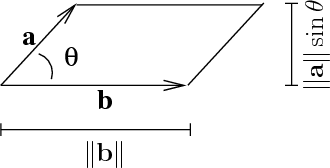# Math Insight

### Image: Area of parallelogramThe area of a parallelogram with spanned by the vectors $\vc{a}$ and $\vc{b}$ is $$\|\vc{a}\|~\|\vc{b}\|\sin\theta,$$ where $\theta$ is the angle between $\vc{a}$ and $\vc{b}$.

Image file: area_parallelogram.png